# Infinite trees of matroids

Welcome back to this series of posts about infinite matroids. Up to this point we’ve seen what infinite matroids are, and we’ve started to understand the notion a little by looking at a variety of examples. Next we will look at how to stick together infinite matroids to build bigger ones. We’ll see that it is possible to stick infinitely many matroids together at once, and this will give us a flexible way to build new infinite matroids by sticking together infinitely many finite matroids.

Let’s start with a familiar way to stick matroids together: via 2-sums. Suppose that we have matroids $M_1$ and $M_2$ with ground sets $E_1$ and $E_2$, and suppose that these ground sets meet in only a single edge $e$. For the sake of simplicity, we’ll assume that $e$ isn’t a loop or a coloop in either matroid. Now we can build a new matroid $M_1 \oplus_2 M_2$ on the ground set $E_1 \triangle E_2$, by taking the circuits to be sets of the following 3 kinds:

• circuits of $M_1$ not containing $e$
• circuits of $M_2$ not containing $e$
• sets of the form $C_1 \cup C_2 -e$, where $C_1$ and $C_2$ are circuits of $M_1$ and $M_2$ respectively, both containing $e$.

These three kinds of circuit are illustrated below: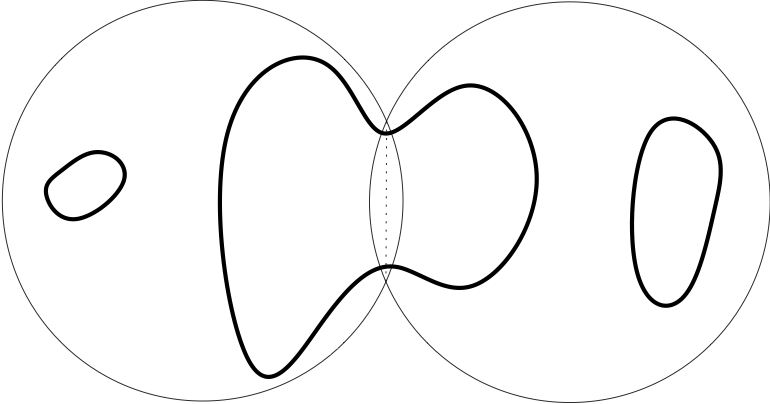This operation is associative, in the sense we might expect: suppose we have 3 matroids $M_1$, $M_2$ and $M_3$ on ground sets $E_1$, $E_2$ and $E_3$. Suppose that $E_1 \cap E_2$ and $E_2 \cap E_3$ each contain just a single edge, and that $E_1 \cap E_3$ is empty. Then the two matroids $M_1 \oplus_2 (M_2 \oplus_2 M_3)$ and $(M_1 \oplus_2 M_2) \oplus_2 M_3$ are equal. This is easy to see by considering the six kinds of circuits which can arise in the sum, as shown here: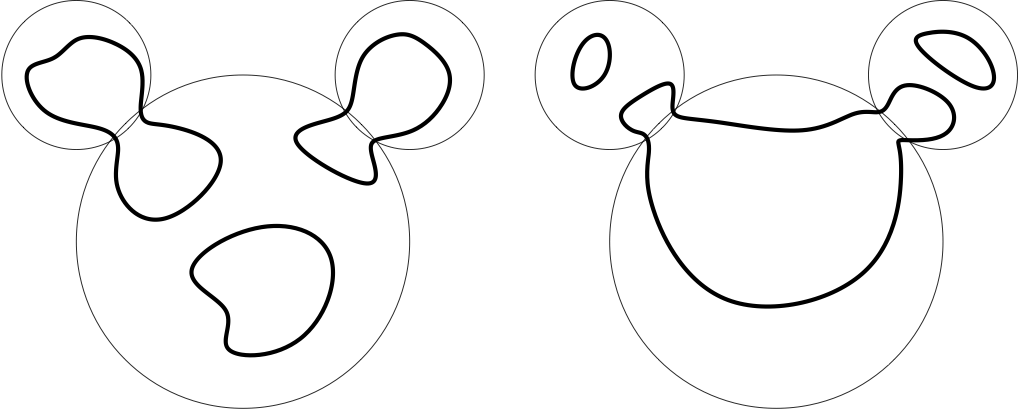Usually associativity means that it doesn’t matter how we bracket a list of things that we want to add together, but in the case of 2-sums the geometry is a little different. The kind of configuration we might want to stick together with 2-sums in general is not a list of matroids, but rather a tree of matroids. More precisely, suppose we have a finite tree $T$, and that for each node $t$ of $T$ we have a matroid $M_t$ with ground set $E_t$. Suppose further that for distinct nodes $t$ and $t’$ of $T$ the intersection $E_t \cap E_{t’}$ either consists of a single edge $e_{tt’}$, if $t$ and $t’$ are neighbours in $T$, or else is empty. Let’s call a structure like this a finite tree of matroids. Then there is an unambiguously defined 2-sum of our tree of matroids, whose ground set is the union of all the sets $E_t$ but without the gluing edges’ $e_{tt’}$. A typical circuit in such a 2-sum of a tree is shown here: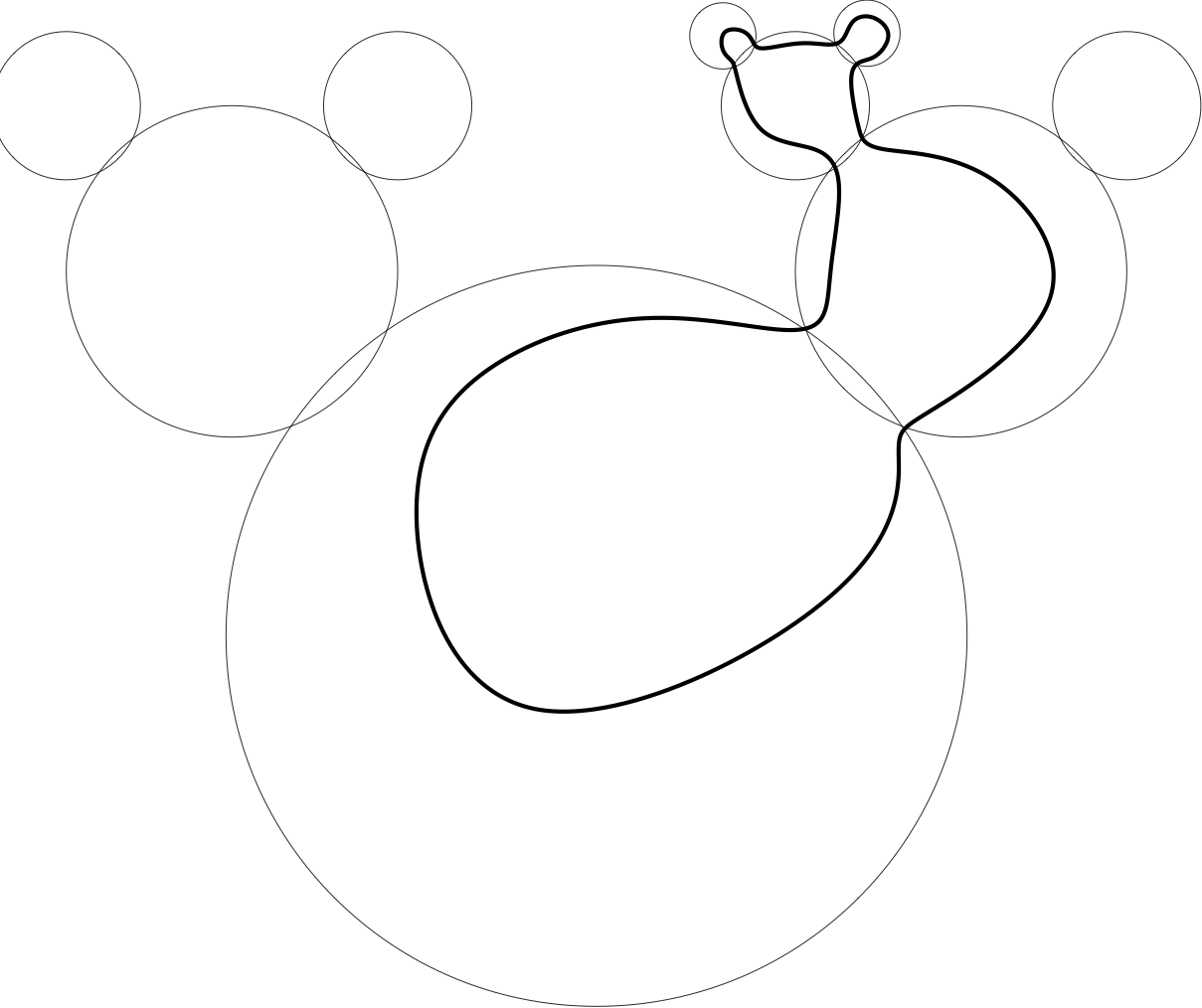More precisely, each circuit of the 2-sum is supported on some subtree $S$ of $T$. It is determined by a choice, for each node $t$ of $S$, of a circuit $C_t$ of $M_t$. This circuit $C_t$ should contain precisely those gluing edges $e_{tt’}$ of $M_t$ such that $t’$ is also in $S$. Given such a subtree $S$ and such a family of circuits $C_t$, we get a circuit of the 2-sum whose edges are the non-gluing edges of the circuits $C_t$.

All of this works just as well for sticking together infinite matroids as for finite ones. But the real power of this technique for building new infinite matroids comes when we consider 2-sums of infinitely many matroids at once.

So what happens if we try to stick together an infinite tree of matroids in the way outlined above? To understand the kinds of difficulty which can arise, let’s first of all consider the simplest possible infinite tree: an infinite ray. We certainly want to allow circuits of the following kind: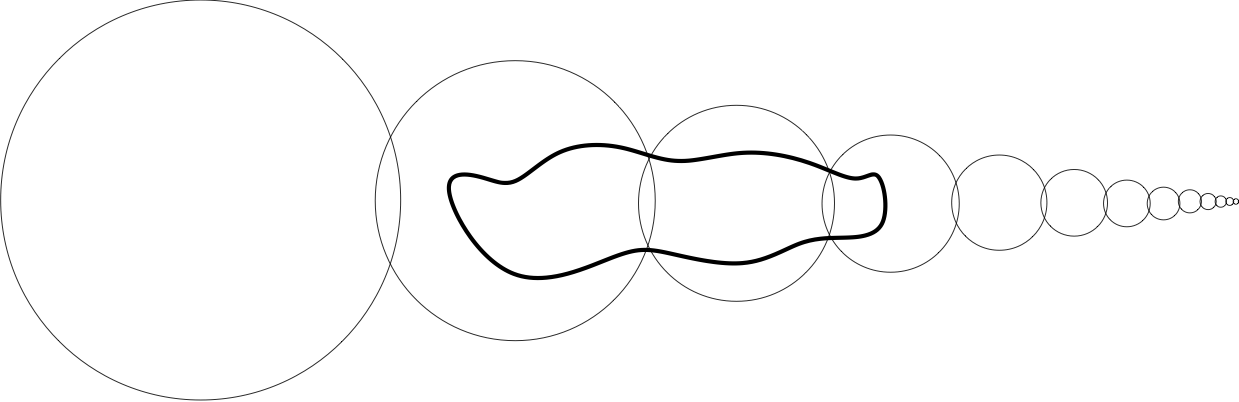But what about ones which go all the way to the end of the ray: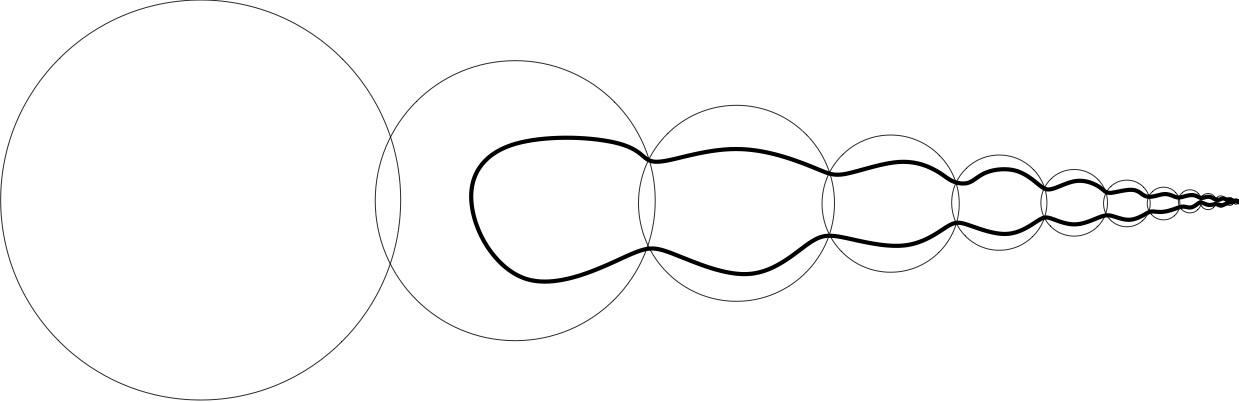Should we allow them?

It turns out that if we allow them, then we get an infinite matroid. But we also get a different infinite matroid by banning them: by only allowing those circuits whose underlying tree $S$ is finite. So we have two different options for how to build a matroid by sticking together an infinite ray of matroids. We say that the circuits are allowed to use the end of the ray in the first of these matroids, but not in the second.

We could just decide to fix one of these two as the correct’ 2-sum of the ray of matroids, but it turns out that that would be a bad idea. To see why, we should consider the interaction of 2-sums with duality. If we take the dual of the 2-sum of 2 matroids, then it turns out to be the same as the 2-sum of their duals. It follows that the same is true for finite trees of matroids: the dual of the 2-sum of a tree of matroids is the 2-sum of the duals of those matroids. This is certainly a property which we would like to keep for infinite 2-sums.

Now suppose that we have a ray of matroids, and we take the variant of the 2-sum where the circuits are not allowed to use the end, then take the dual of that 2-sum. What we get is the 2-sum of the duals of the matroids, but this time in the version where the circuits are allowed to use the end. So these two different constructions of the 2-sum of a ray are dual to each other, and we shouldn’t privilege either of them.

Formally, we take the decision about whether the circuits should be allowed to use the end of the ray to be part of the data used to specify the sum, in addition to the choices of the matroids lying along the ray. More generally, suppose that we have an infinite tree of matroids. Then in order to specify a 2-sum for this tree of matroids, we must decide, for each end of the tree, whether the circuits are allowed to use that end. Here the ends of the tree can be identified with the rays from a fixed root.

More precisely, suppose that we have a (possibly infinite) tree $T$, and that for each node $t$ of $T$ we have a matroid $M_t$ with ground set $E_t$. Suppose further that for distinct nodes $t$ and $t’$ of $T$ the intersection $E_t \cap E_t’$ either consists of a single edge $e_{tt’}$, if $t$ and $t’$ are neighbours in $T$, or else is empty. Suppose further that we have a subset $\Psi$ of the set of ends of $T$, which we intend to be the set of ends which we will allow circuits to use. Then we might hope to define the 2-sum of this tree of matroids as follows:

Each circuit of the 2-sum will be supported on some subtree $S$ of $T$, such that all rays in $S$ go to ends in $\Psi$. The circuit is determined by a choice, for each node $t$ of $S$, of a circuit $C_t$ of $M_t$. This circuit $C_t$ should contain precisely those gluing edges $e_tt’$ of $M_t$ such that $t’$ is also in $S$. Given such a subtree $S$ and such a family of circuits $C_t$, we get a circuit of the 2-sum whose edges are the non-gluing edges of the circuits $C_t$.

So the circuits would look something like this: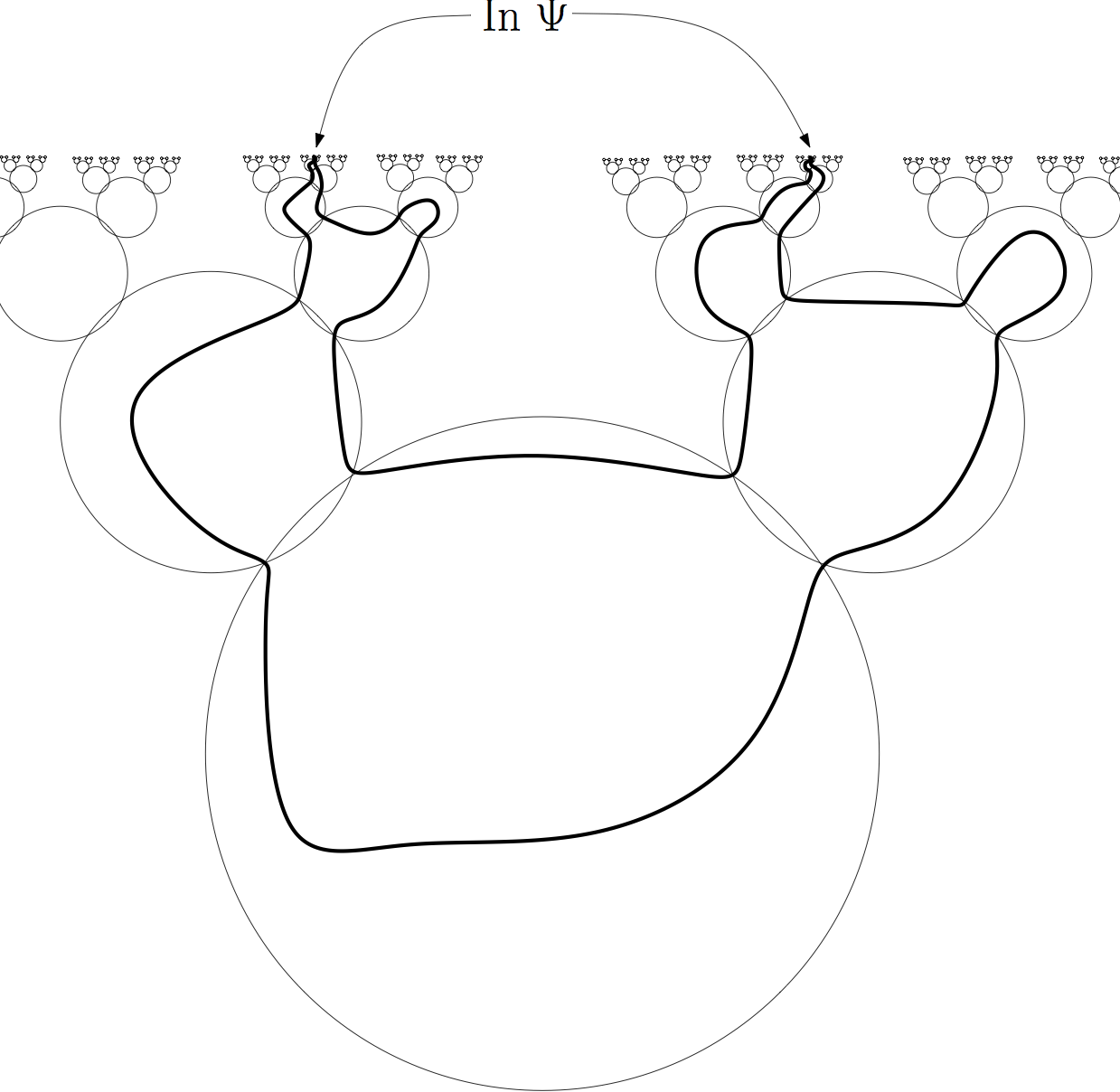Unfortunately, this construction does not always give a matroid. The reason why not is related to some tricky set-theoretical issues, so we will not discuss it here. But due to a very deep and beautiful set-theoretic result called Borel Determinacy, we know that this construction will give a matroid if the set $\Psi$ is topologically nice enough (if it is a Borel set) [BC15].

There are also certain infinite trees of matroids such that the above construction will give a matroid for any set $\Psi$ of ends. This has the immediate consequence that there are a lot of infinite matroids – as many as there could possibly be. A matroid is determined by a set of subsets of its ground set, so there could be no more than $2^{2^{\aleph_0}}$ matroids on a countable set. And in fact, there are that many non-isomorphic matroids on a countable set, because there are that many possibilities for the set $\Psi$.

This means that when an infinite matroid is constructed from a tree of matroids together with a set $\Psi$ of ends, most of the information is encoded in the set $\Psi$ – if the matroids in the tree are finite, then there are only $2^{\aleph_0}$ possibilities for the tree of matroids, but there are $2^{2^{\aleph_0}}$ possibilities for $\Psi$. This huge reservoir of extra information hidden at the ends means that countable matroids behave in many ways like uncountable graphs. For example, the constructions which show that infinite graphs in general are not well-quasi-ordered under the minor relation already work for countable matroids, even though they do not work for countable graphs [BC15].

The construction we are considering also plays a key role for the reconstruction of infinite matroids from their decompositions into 3-connected parts. It is known that any finite matroid is canonically expressible as a 2-sum of a finite tree of matroids, each of which is either 3-connected or else consists of a single circuit or cocircuit [CE80, S80]. This result extends directly to infinite matroids: any matroid can be canonically expressed as a 2-sum (in the above sense) of a tree of matroids, each of which is either 3-connected or else consists of a single circuit or cocircuit [ADP15, BC16]. As with the finite result, this implies that it often suffices to prove a result only for 3-connected matroids in order to be able to deduce it for all matroids.

The construction we have considered was based on the 2-sum, one of the simplest possible ways to stick matroids together. Next time, we shall see that similar ideas work to give infinitary versions of other more complicated ways of sticking matroids together, and the surprisingly close connections between these infinitary sums and the matroids naturally arising in infinite graphs.

[ADP15] E. Aigner-Horev, R. Diestel, L. Postle. The structure of 2-separations of infinite matroids, to appear in JCTB, available here.
[BC15] N. Bowler and J. Carmesin, Infinite Matroids and Determinacy of Games, submitted to LMS, available here.
[BC16] N. Bowler and J. Carmesin, The ubiquity of Psi-matroids, preprint, available here.
[CE80] W. H. Cunningham and J. Edmonds, A combinatorial decomposition theory, Canad. J. Math. 32 (1980), no. 3, 734–765.
[S80] P. D. Seymour, Decomposition of regular matroids, J. Combin. Theory Ser. B 28 (1980), no. 3, 305–359.

This entry was posted in Matroids by Nathan Bowler. Bookmark the permalink.I'm working at the university of Hamburg on infinite matroid theory.

## 7 thoughts on “Infinite trees of matroids”

1.Patrick Tam on said:

Hello, I’m interested in constructions that give matroids. Under the axioms for infinite matroids, the dual of a matroid is a matroid. I’m interested in knowing whether there’s a more general version of this statement. Let $M=(E,L)$ and $N=(E,J)$ be two matroids with a common ground set. Suppose that $L \subset J$. I define the $N-M=(E,K)$ where $S \in K$ if there exists a base $F$ in $N$ and a base $B$ in $M$ such that $B \subset F$ and $S \subset F \setminus B$. Is $N-M$ necessarily a matroid? If $N=(E,2^{E})$, then $N-M$ is the dual of $M$.

I also sent you an email applying a specific instance of this construction to study the problem of whether every nearly finitary matroid is $k$-nearly finitary.

•Nathan Bowler on said:

Hi Patrick,

thanks for your comment. It seems to me that, at least on a finite ground set, the construction you suggest should give a matroid, namely the dual of the union of N with the dual of M. This uses the matroid union theorem.

2.Patrick Tam on said:

Hello, I’ve been thinking about Psi-matroids and I think I might have a way to generalize them. Let G be a locally finite graph that contains no subdivision of the bean graph or the dominated ladder. Given a set Psi of ends of G, lets consider a partition P of Psi. I want to add a point at infinite for each element p of P. I want to consider double rays between ends in the same component of P to be topological circuits of G. Ideally, I want my construction to correspond to the algebraic cycle matroid when Psi consists of all ends of G and P is the singleton partition of Psi with only one component. I want it to correspond to the topological cycle matroid when Psi consists of all ends of G and P is the partition where every component is a singleton set. I’m not sure when this construction gives a matroid.

•Nathan Bowler on said:

Hi again Patrick,

that sounds like a really good idea. As you suggest, the algebraic cycle matroid looks like it is coming from the singleton partition in this way. It would be great to hear if you make any progress with this idea. For example, which bipartitions of the set of all ends induce infinite matroids in this way? What do you think?

•Patrick Tam on said:

I’m not sure but I’m guessing each component of the bipartition has to be Borel measurable based on what happens with ordinary Psi-matroids.

•Nathan Bowler on said:

That’s what I would have expected to be the right condition too, but thinking about it some more I’m not so sure. For example, lets say we partition the set of ends of an infinite binary tree into two dense Borel sets. Then there’s no cut of the tree separating those two sets from each other, so it’s not clear what the cocircuits of the corresponding matroid could be. So it seems that in this case we might not get a matroid (of course this isn’t a proof). What do you think?

•Patrick Tam on said:

I suppose it might be easier to find a nice sufficient condition by strengthening that condition then. Maybe require the existence of a cut separating the two sets. I’m not sure what kind of condition would be necessary and sufficient.

This site uses Akismet to reduce spam. Learn how your comment data is processed.